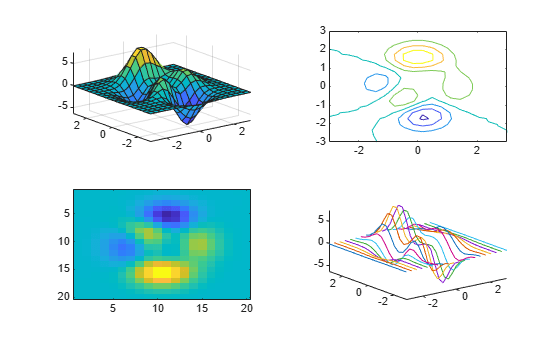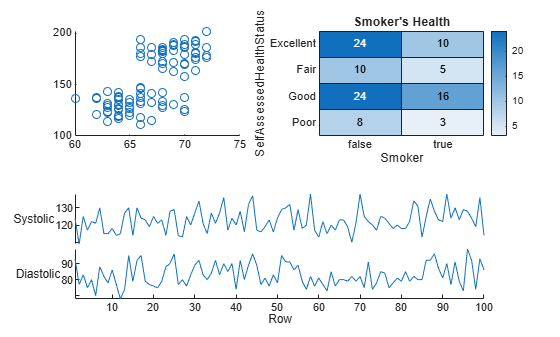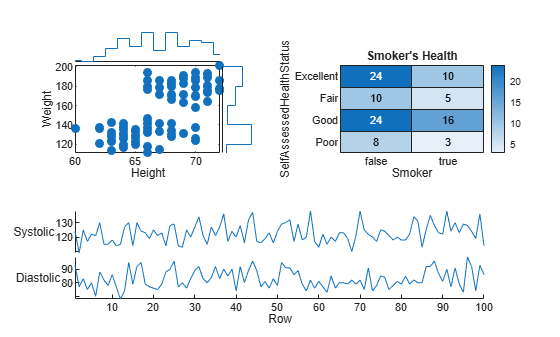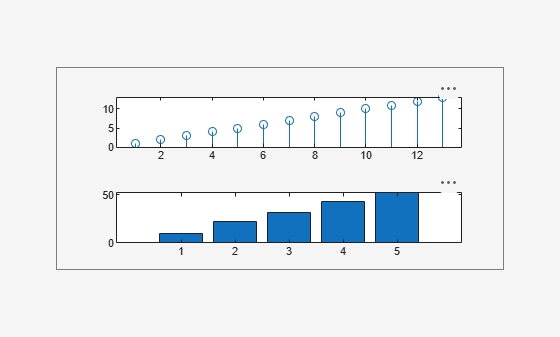# nexttile

Create axes in tiled chart layout

## Syntax

``nexttile``
``nexttile(span)``
``nexttile(tilenum)``
``nexttile(tilenum,span)``
``nexttile(t,___)``
``ax = nexttile(___)``

## Description

example

````nexttile` creates an axes object and places it into the next empty tile of the tiled chart layout that is in the current figure. If there is no layout in the current figure, then `nexttile` creates a new layout and configures it using the `'flow'` tile arrangement. The resulting axes object is the current axes, so the next plotting command can plot into it.```

example

````nexttile(span)` creates an axes object that spans across multiple rows or columns. Specify `span` as a vector of the form `[r c]`. The axes spans `r` rows by `c` columns of tiles. The upper left corner of the axes is positioned in the upper left corner of the first empty `r`-by-`c` region.```

example

````nexttile(tilenum)` assigns the current axes to be the axes or chart in the specified tile. Specify `tilenum` as the tile number. The tile numbers start at `1` and increase from left to right and from top to bottom. In some cases, `nexttile` creates a new axes object: When the specified tile is empty, `nexttile` creates an axes object in that tile.When the specified tile contains part of an axes or chart object, but not its upper left corner, `nexttile` replaces the existing axes or chart object. For example, if `tilenum` refers to a tile in the middle of an axes object that spans multiple tiles, `nexttile` replaces the existing axes object with a new one in the tile you specify. ```

example

````nexttile(tilenum,span)` creates an axes object that spans across multiple rows or columns, starting at the tile specified by `tilenum`. If the tile you specify is occupied by an axes or chart object, `nexttile` either makes that object the current axes or replaces it: If the existing axes or chart object spans across the same set of tiles as the `tilenum` and `span` arguments specify, then `nexttile` makes that axes or chart object the current axes.If the existing axes or chart object spans across a different set of tiles than the `tilenum` and `span` arguments specify, then `nexttile` replaces the existing axes or chart object with a new axes object using the new `tilenum` and `span` values. ```

example

````nexttile(t,___)` operates on the tiled chart layout specified by `t`. Specify `t` before all other input arguments. This syntax is useful when you are working with multiple layouts, or when the layout is in a panel or tab instead of a figure.```

example

````ax = nexttile(___)` returns the axes object. Use `ax` to set properties on the axes. You can also pass `ax` as an input argument to other graphics functions that operate on an axes object. For example, you can call the `colormap` or `colororder` function to change the color scheme for the axes.```

## Examples

collapse all

If a tiled chart layout does not already exist, `nexttile` creates one.

Create four coordinate vectors: `x`, `y1`, `y2`, and `y3`. Next, call the `nexttile` function to create a tiled chart layout and an axes object in the first tile. Then plot `y1` in the first tile. This first plot fills the entire layout because `nexttile` creates the layout using the `'flow'` tile arrangement.

```x = linspace(0,30); y1 = sin(x/2); y2 = sin(x/3); y3 = sin(x/4); % Plot into first tile three times nexttile plot(x,y1)```Create a second tile and axes, and plot into the axes.

```nexttile plot(x,y2)```Repeat the process to create a third plot.

```nexttile plot(x,y3)```Repeat the process to create a fourth plot. This time, plot all three lines in the same axes by calling `hold on` after plotting `y1`.

```nexttile plot(x,y1) hold on plot(x,y2) plot(x,y3) hold off```Call the `tiledlayout` function to create a `2`-by-`2` tiled chart layout, and call the `peaks` function to get the coordinates of a predefined surface. Create an axes object in the first tile by calling the `nexttile` function. Then call the `surf` function to plot into the axes. Repeat the process using different plotting functions for the other three tiles.

```tiledlayout(2,2); [X,Y,Z] = peaks(20); % Tile 1 nexttile surf(X,Y,Z) % Tile 2 nexttile contour(X,Y,Z) % Tile 3 nexttile imagesc(Z) % Tile 4 nexttile plot3(X,Y,Z)```Call the `tiledlayout` function to create a `2`-by-`1` tiled chart layout. Call the `nexttile` function with an output argument to store the axes. Then plot into the axes, and set the x- and y-axis colors to red. Repeat the process in the second tile.

```t = tiledlayout(2,1); % First tile ax1 = nexttile; plot([1 2 3 4 5],[11 6 10 4 18]); ax1.XColor = [1 0 0]; ax1.YColor = [1 0 0]; % Second tile ax2 = nexttile; plot([1 2 3 4 5],[5 1 12 9 2],'o'); ax2.XColor = [1 0 0]; ax2.YColor = [1 0 0];```Define `scores` and `strikes` as vectors containing bowling league data over four games. Then create a tiled chart layout and display three plots showing the number of strikes for each team.

```scores = [444 460 380 387 366 500 365 451 611 548 412 452]; strikes = [9 6 5 6 4 8 4 7 16 10 9 8]; t = tiledlayout('flow'); % Team 1 nexttile plot([1 2 3 4],strikes(:,1),'-o') title('Team 1 Strikes') % Team 2 nexttile plot([1 2 3 4],strikes(:,2),'-o') title('Team 2 Strikes') % Team 3 nexttile plot([1 2 3 4],strikes(:,3),'-o') title('Team 3 Strikes')```Call the `nexttile` function to create an axes object that spans two rows by three columns. Then display a bar graph in the axes with a legend, and configure the axis tick values and labels. Call the `title` function to add a tile to the layout.

```nexttile([2 3]); bar([1 2 3 4],scores) legend('Team 1','Team 2','Team 3','Location','northwest') % Configure ticks and axis labels xticks([1 2 3 4]) xlabel('Game') ylabel('Score') % Add layout title title(t,'April Bowling League Data')```To span an axes object from a specific location, specify the tile number and the span value.

Define `scores` and `strikes` as vectors containing bowling league data over four games. Then create a `3`-by-`3` tiled chart layout and display five bar graphs showing the number of strikes for each team.

```scores = [444 460 380 388 389 387 366 500 467 460 365 451 611 426 495 548 412 452 471 402]; strikes = [9 6 5 7 5 6 4 8 10 7 4 7 16 9 9 10 9 8 8 9]; t = tiledlayout(3,3); % Team 1 nexttile bar([1 2 3 4],strikes(:,1)) title('Team 1 Strikes') % Team 2 nexttile bar([1 2 3 4],strikes(:,2)) title('Team 2 Strikes') % Team 3 nexttile bar([1 2 3 4],strikes(:,3)) title('Team 3 Strikes') % Team 4 nexttile bar([1 2 3 4],strikes(:,4)) title('Team 4 Strikes') % Team 5 nexttile(7) bar([1 2 3 4],strikes(:,5)) title('Team 5 Strikes')```Display a larger plot with a legend. Call the `nexttile` function to place the upper left corner of the axes in the fifth tile, and span the axes across two rows by two columns of tiles. Plot the scores for all the teams. Configure the x-axis to display four ticks, and add labels to each axis. Then add a shared title at the top of the layout.

```nexttile(5,[2 2]); plot([1 2 3 4],scores,'-.') labels = {'Team 1','Team 2','Team 3','Team 4','Team 5'}; legend(labels,'Location','northwest') % Configure ticks and axis labels xticks([1 2 3 4]) xlabel('Game') ylabel('Score') % Add layout title title(t,'April Bowling League Data')```Create a 1-by-2 tiled chart layout. In the first tile, display a geographic plot containing a line that connects two cities on a map. In the second tile, create a scatter plot in polar coordinates.

```tiledlayout(1,2) % Display geographic plot nexttile geoplot([47.62 61.20],[-122.33 -149.90],'g-*') % Display polar plot nexttile theta = pi/4:pi/4:2*pi; rho = [19 6 12 18 16 11 15 15]; polarscatter(theta,rho)```One of the ways that the `nexttile` output argument is useful is when you want to adjust the content in a previous tile. For example, you might decide to reconfigure the colormap used in a previous plot.

Create a 2-by-2 tiled chart layout. Call the `peaks` function to get the coordinates for a predefined surface. Then create a different plot of the surface in each tile.

```tiledlayout(2,2); [X,Y,Z] = peaks(20); % Tile 1 nexttile surf(X,Y,Z) % Tile 2 nexttile contour(X,Y,Z) % Tile 3 nexttile imagesc(Z) % Tile 4 nexttile plot3(X,Y,Z)```To change the colormap in the third tile, get the axes in that tile. Call the `nexttile` function by specifying the tile number, and return the axes output argument. Then pass the axes to the `colormap` function.

```ax = nexttile(3); colormap(ax,cool)```Load the `patients` data set and create a table from a subset of the variables. Then create a `2`-by-`2` tiled chart layout. Display a scatter plot in the first tile, a heatmap in the second tile, and a stacked plot across the bottom two tiles.

```load patients tbl = table(Diastolic,Smoker,Systolic,Height,Weight,SelfAssessedHealthStatus); tiledlayout(2,2) % Scatter plot nexttile scatter(tbl.Height,tbl.Weight) % Heatmap nexttile heatmap(tbl,'Smoker','SelfAssessedHealthStatus','Title','Smoker''s Health'); % Stacked plot nexttile([1 2]) stackedplot(tbl,{'Systolic','Diastolic'});```Call `nexttile`, and specify the tile number as `1` to make the axes in that tile the current axes. Replace the contents of that tile with a scatter histogram.

```nexttile(1) scatterhistogram(tbl,'Height','Weight');```Create a panel in a figure. Then create a tiled chart layout `t` in the panel by specifying the panel object as the first argument to the `tiledlayout` function. By default, `nexttile` looks for the layout in the figure. However the layout is in a panel instead of a figure, so you must specify `t` as an input argument when you call `nexttile`.

```p = uipanel('Position',[.1 .2 .8 .6]); t = tiledlayout(p,2,1); % Tile 1 nexttile(t) stem(1:13) % Tile 2 nexttile(t) bar([10 22 31 43 52])```Occasionally, you might need to create the axes manually before calling a plotting function. When you create the axes, specify the `parent` argument as the tiled chart layout. Then position the axes by setting the `Layout` property on the axes.

Create a tiled chart layout `t` and specify the `'flow'` tile arrangement. Display a plot in each of the first three tiles.

```t = tiledlayout('flow'); nexttile plot(rand(1,10)); nexttile plot(rand(1,10)); nexttile plot(rand(1,10));```Create a geographic axes object `gax` by calling the `geoaxes` function and specify `t` as the parent argument. By default, the axes goes into the first tile, so move it to the fourth tile by setting `gax.Layout.Tile` to `4`. Span the axes across a `2`-by-`3` region of tiles by setting `gax.Layout.TileSpan` to `[2 3]`.

```gax = geoaxes(t); gax.Layout.Tile = 4; gax.Layout.TileSpan = [2 3];```Call the `geoplot` function. Then configure the map center and zoom level for the axes.

```geoplot(gax,[47.62 61.20],[-122.33 -149.90],'g-*') gax.MapCenter = [47.62 -122.33]; gax.ZoomLevel = 2;```## Input Arguments

collapse all

Tile number, specified as a positive whole number. The tile numbers start at `1` and increase from left-to-right and top-to-bottom.

If the tile is empty, `nexttile` places an axes object into that tile. If the tile contains an axes or chart object, then that object becomes the current axes, so the next plotting command can plot into it.

Tile span, specified as a vector of the form `[r c]`, where `r` and `c` are positive whole numbers. Use this argument to make the axes span `r` rows by `c` columns of tiles in the layout.

If you specify the `span` argument without the `tilenum` argument, `nexttile` places the upper left corner of the axes in the upper left corner of the first empty `r`-by-`c` region in the layout.

However, if you specify both the `tilenum` and `span` arguments, `nexttile` places the upper left corner of the axes in the upper left corner of the tile specified by `tilenum`. For example, the large axes on the right side of this `3`-by-`4` layout has a tile number of `2` and a span of `[2 3]`.`TiledChartLayout` object to place the axes into. This argument is useful when you are working with multiple layouts, or when the layout is in a panel or tab instead of a figure. When you do not specify `t`, `nexttile` looks for the layout in the current figure.